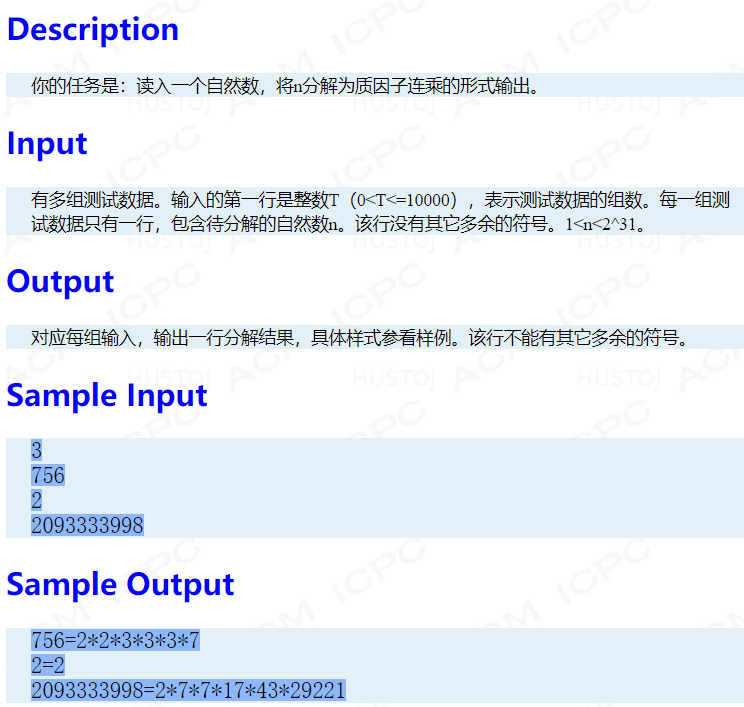`````` #include <stdio.h>
#include <math.h>
int isPrime(int k)
{
int i;
for (i = 2; i <= sqrt(k); i++)
{
if (k % i == 0)
return 0;
}
return 1;
}
int getFactors(int factors[], int n)
{
int cnt = 0;
int k;
for (k = 2; k <= n; k++)
{
if (isPrime(k) && n % k == 0)
{
factors[cnt++] = k;
n /= k;
while (n % k == 0)
{
factors[cnt++] = k;
n /= k;
}
}
}
return cnt;
}
int output(int factors[], int cnt)
{
int i;
printf("%d", factors);
for (i = 1; i < cnt; i++)
printf("*%d", factors[i]);
putchar('\n');
return 0;
}
int main()
{
int factors;
int T, n, cnt, j;
scanf("%d", &T);
for (j = 0; j < T; j++)
{
scanf("%d", &n);
cnt = getFactors(factors, n);
printf("%d=", n);
output(factors, cnt);
}
return 0;
}

``````

1个回答tilblackout AC了,问题在于k <= n改成k<=sqrt(n) 但是如果n本身是最后一个因子就不输出 只要最后判断一下 if(n!=1) factors[cnt++] = n;就好了tilblackout 好像真不是判断素数的问题 我发现不需要判断素数 因为从2开始 如果有不是素数的已经被分解了。我把那个函数去了下面直接if (n % k == 0) 仍然超时。说明应该真的不是判断素数的问题。tilblackout 抱歉看错了..不过素数计算效率怎为什么会低呢。需要怎么改进。tilblackout 我觉得你在胡说..这个地方我改过floor(sqrt(n)+0.5) 一样错 而且我说了是time limit exceed 如果问题出在这里也是wrong answer